Latest Banking jobs   »   v

# Quantitative Aptitude Quiz For Bank of Baroda AO 2023 -11th April

Directions (1-8): Find the approximate value of (?) in the following questions.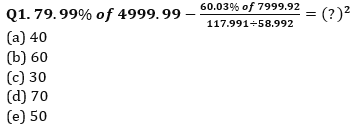Q2. 70.03% of 900.003-(34.991 of 16.02÷4.999)= ?+193.021
(a) 445
(b) 325
(c) 380
(d) 415
(e) 480
Q3. ((46.03% of 2999.98)÷68.996 of (10.01% of 20))= ?
(a) 50
(b) 40
(c) 20
(d) 30
(e) 10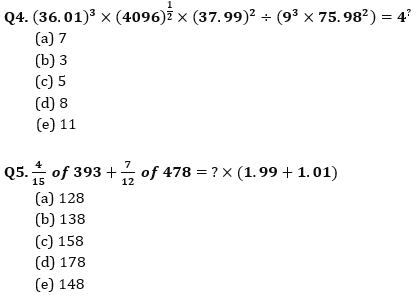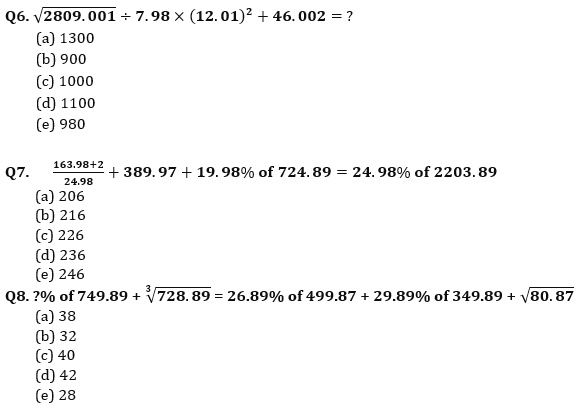Directions (9-12): Find the wrong number in the following number series
Q9. 190, 210, 266, 358, 486, 646, 850
(a) 646
(b) 850
(c) 486
(d) 190
(e) 210

Q10. 15, 50, 160, 370, 709, 1208, 1904
(a) 15
(b) 50
(c) 370
(d) 1208
(e) 15

Q11. 120, 170, 251, 367, 522, 720, 990
(a) 120
(b) 990
(c) 522
(d) 367
(e) 251
Q12. 32, 75, 144, 245, 384, 569, 800
(a) 144
(b) 569
(c) 245
(d) 75
(e) 32

Direction (13 – 15): in the following questions, a series is given in which one term is wrong with which another series started. You have to find the wrong term in the given series then starting from that, find 5th term of new series so formed.
Example – 1, 2, 5, 21, 88, 445, 2676
Pattern of above series –
1 ×1+1=2
2 ×2+2=6
6 ×3+3=21
21 ×4+4=88
88 ×5+5=445
445 ×6+6=2676
So, wrong number = 5
So, new series
5 ×1+1=6
6 ×2+2=14
14 ×3+3=45
45 ×4+4=184
184 ×5+5=925
925 ×6+6=5556
So, 5th term of new series = 184

Q13. 3, 2, 6, 15, 60, 315, 1896
(a) 59
(b) 42816
(c) 7135
(d) 357
(e) 1432
Q14. 993, 850, 750, 688, 653, 638, 635
(a) 410
(b) 445
(c) 395
(d) 392
(e) 508
Q15. 20, 30, 50, 90, 160, 330, 650
(a) 230
(b) 470
(c) 310
(d) 190
(e) None of these

Solutions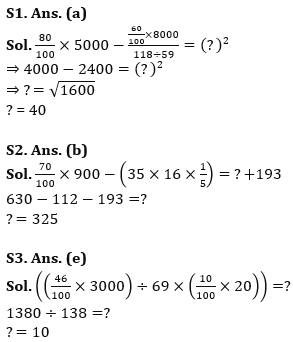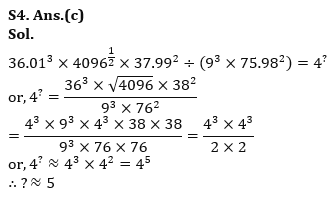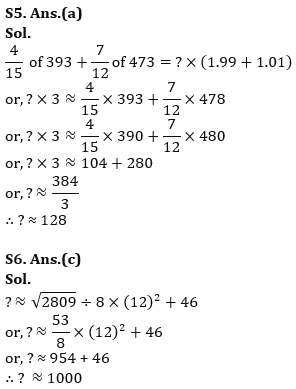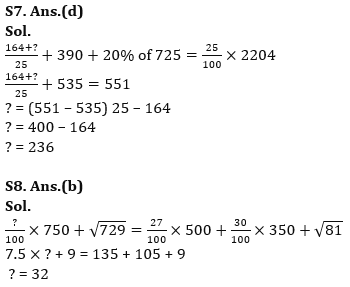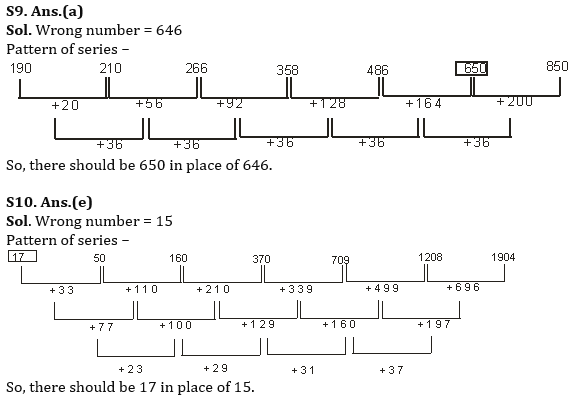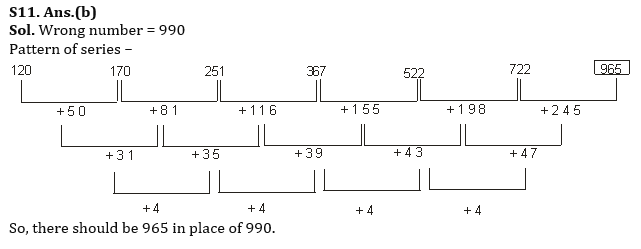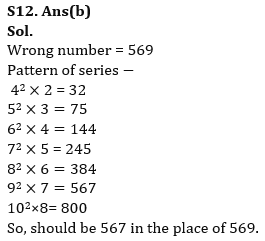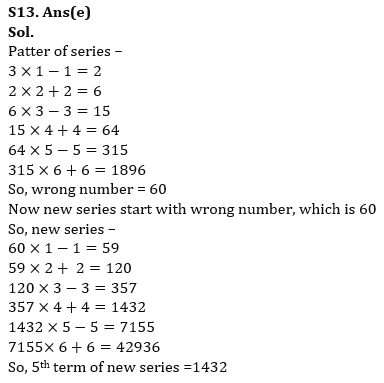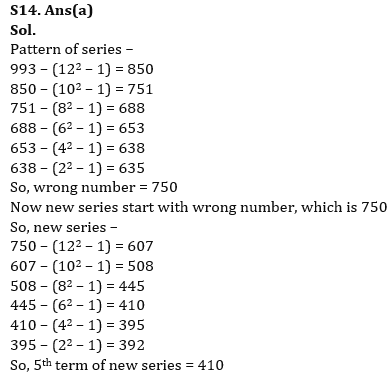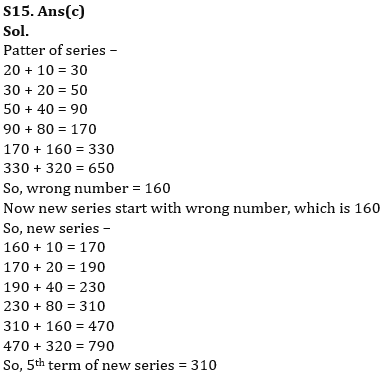## FAQs

### What is the selection process of Bank of Baroda AO 2023?

The selection process of Bank of Baroda AO consists of Online exam and Interview

#### Congratulations!Union Budget 2023-24: Free PDF